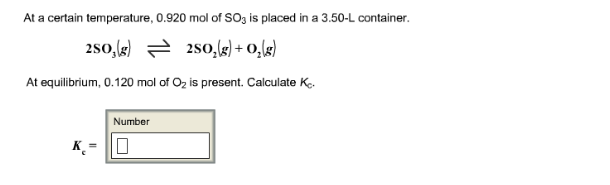# Problem: At a certain temperature, 0.920 mol of SO3 is placed in a 3.50-L container. 2SO3(g) ⇌ 2SO2(g) + O2(g) At equilibrium, 0.120 mol of O2 is present. Calculate Kc.

🤓 Based on our data, we think this question is relevant for Professor Mathias' class at BROOKLYN CUNY.

###### FREE Expert Solution###### Problem Details

At a certain temperature, 0.920 mol of SO3 is placed in a 3.50-L container. 2SO3(g) ⇌ 2SO2(g) + O2(g) At equilibrium, 0.120 mol of O2 is present. Calculate Kc